# Feature Engineering for Categorical Data

## Several Useful Tricks in Addition to One-Hot Encoding

In machine learning, features refer to the inputs to machine learning models or numerical representations of raw data. There two main types of features in tabular data: numerical feature and categorical feature. Feature engineering is a process of extracting features from raw data and transforming them into suitable formats for the machine learning models. For numerical features, the most frequently used feature engineerings are log transformation, standardization, and normalization. For categorical features, we have many options to transform them into numbers, but when and how to use those methods? This article is all about implementing feature engineering for categorical features.

Let me introduce the dataset we used for illustration before we dig into the techniques: in-vehicle coupon recommendation. This dataset was collected via a survey on Amazon Mechanical Turk by Wang et al. (2017). The survey describes different driving scenarios including the destination, current time, weather, passengers, etc., and then asks the person whether he or she would like to accept the coupon if he or she is the driver. Nearly all features in the in-vehicle coupon recommendation dataset are categorical, those features can be divided into three types: user attributes, contextual attributes, and coupon attributes. For simplicity, I will skip the detailed introduction about those features, for more information about the original dataset, one can look at the dataset link above, or check my Kaggle notebook.

## Ordinal Encoding

Just as its name indicates, this method is only suitable for ordinal data. Ordinal data is one kind of categorical data that has inner order in it. For example rank, age range, and income range. In our dataset, we have those ordinal columns: Restaurant20To50, RestaurantLessThan20, CarryAway, CoffeeHouse, Bar, age, and income. Below is my implementation of ordinal encoding on those columns:

## One-Hot Encoding

Nominal data is the other kind of categorical data, for nominal variables, the simplest encoding method is one-hot encoding. If one variable has three categories, then the one-hot encoding will transform it into three new categories. For example, a variable named color has three categories: red, green, and blue. After one-hot encoding, the new variables should be like this:

The main drawback of one-hot encoding is the increased number of dimensions. If you have a variable with many categories, after one-hot encoding, the dataset will be more sparse than before. One-hot encoding can be implemented by `sklearn.preprocessing.OneHotEncoder`. Here is an example of implementing feature engineering for mixed data types with `sklearn.Pipeline` and `ColumnTransformer`.

## Frequency Encoding

Frequency encoding is another technique for nominal data, it encodes the frequency of a category, and thus will not increase the number of dimensions in the dataset. Here is a simple example:

After frequency encoding, the original dataset will be:

The categories are replaced by the frequency that category appeared in this variable. Notice that when some categories have the same frequencies just like this example, we did lose the information of the original data. Below is the implementation of frequency encoding referred to Bhavika’s code.

## K Fold Target Encoding

Target encoding is one of the magic methods in feature engineering for categorical data, the basic idea is using a statistic of categories with respect to the target to encode the original categories. Here is a simple example of target encoding. Suppose we have a binary target variable, and three categories: red, green, and blue:

If we use mean target encoding to encode the three colors with the expected value of the target variable, we will get `red_target = 0.5`, `green_target = 0.25`, `blue_target = 0.4`.

As for the disadvantages of target encoding, the first drawback is just like ordinal frequency encoding — not robust. Information can be lost during this transformation especially when the expected values of the target are very close for many categories. Another disadvantage is the risk of data leakage due to the involvement of the target variable in the calculation. To alleviate this problem, the k fold technique can be used to crossly calculate the expected value of the target variable. Here is an article from Pourya, which clearly explained the mechanism of k fold target encoding and provided code to implement it. Based on his work, I added some docstrings and comments to make the code more readable:

Here is my code to implement them for both training and testing data:

## Feature Expansion Using K-Prototypes Clustering

In addition to encoding methods, there are other feature engineering techniques like dimension reduction and feature expansion. In this article, we are going to introduce one of the efficient feature expansion methods for mixed data type — feature expansion using k-prototypes clustering.

For numerical data, the k-means algorithm is the most frequently used clustering method for feature expansion. Compared to hierarchical agglomerative clustering, k-means is more efficient since it is of linear complexity while hierarchical clustering is of quadratic complexity which makes it costly for large datasets.

To implement feature expansion with k-means clustering, we just simply add the clustering label as a new feature. If the target information is available, we can also include it to improve the clustering procedure. By doing so, the target information will be leaked into the clustering membership, but in most cases, we don’t need to concern about it since the lossy compression of the clustering algorithm will have abstracted away some of the target information. When the dataset is small, k-fold cross-validation can be used to reduce the bias introduced by including the target feature.

However, the k-means algorithm makes no sense for categorical data. To extend the usage of the k-means algorithm, k-modes and k-prototypes algorithm were invented by Huang. Citing from his paper: “The k-modes algorithm uses a simple matching dissimilarity measure to deal with categorical objects, replaces the means of clusters with modes, and uses a frequency-based method to update modes in the clustering process to minimize the clustering cost function. With these extensions, the k-modes algorithm enables the clustering of categorical data in a fashion similar to k-means. The k-prototypes algorithm, through the definition of a combined dissimilarity measure, further integrates the k-means and k-modes algorithms to allow for clustering objects described by mixed numeric and categorical attributes.” Thus, in most cases, k-prototypes is a decent solution for clustering of mixed data.

The Python implementation of the k-modes and k-prototypes algorithm can be found in the kmodes package. To implement the k-prototypes algorithm, the first thing we need to do is find an appropriate k. Silhouette diagram can be used for it. Below is the code to find an appropriate k using our dataset, the code for plotting silhouette diagram is borrowed from Hands-On Machine Learning with Scikit-Learn, Keras, and TensorFlow, 2nd Edition by Aurelien Geron.

To use k-prototypes, we need to point out the column indices of categorical features. After using sklearn’s preprocessor, the column names and indices have changed:

Then we include the target feature, and plot a silhouette diagram for k from 2 to 9:

Silhouette coefficient ranges from -1 to +1, the silhouette score is the mean silhouette coefficient over all the instances within the cluster. A coefficient close to +1 means that the instance is well inside its own cluster and far from other clusters, while a coefficient close to 0 means that it is close to a cluster boundary, and finally a coefficient close to –1 means that the instance may have been assigned to the wrong cluster.

According to the silhouette plot for data plan A, the silhouette score looks good when 𝑘=2,3,4,5 as the red dashed line indicates the average silhouette score. We are picking 𝑘=4as the number of clusters of our k-prototypes clustering.

After picking the number of k, now we can implement the k-prototypes algorithm for feature expansion. The featurizer below is based on the k-means featurizer in Feature Engineering for Machine Learning by Alice Zheng and Amanda Casari, I modified the code to make it suitable for k-prototypes.

Here is how we used it using the in-vehicle coupon dataset:

## Comparison among methods

We applied data in plan A with frequency and target encoding, while data in plan B with one-hot encoding. The feature expanded version is based on plan A. And below is the testing accuracy of the classification by five different basic models. Frequency and target encoded version achieved the highest accuracy on k nearest neighbors classifier, while feature expanded version achieved the highest accuracy on decision tree induction model.

## Summary

In this article, some feature engineering techniques for categorical data are introduced. For more information about the classification task on the in-vehicle coupon dataset and the interactions between those feature engineering methods and machine learning models, please check my kaggle notebook.

Feel free to comment and share your opinions!

--

--

--

## More from Geek Culture

A new tech publication by Start it up (https://medium.com/swlh).

## Data Pre-processing with Data reduction techniques in Python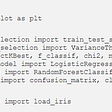## Biological Inspiration of Convolutional Neural Network (CNN)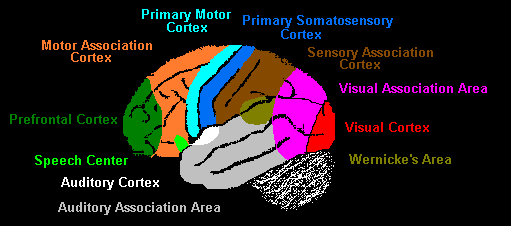## Facial Recognition System with JavaScript## Understanding Performance metrics for Machine Learning Algorithms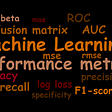## Random Forest Classifier## 9 Types of Performance Evaluation for Classification Machine Learning Modeling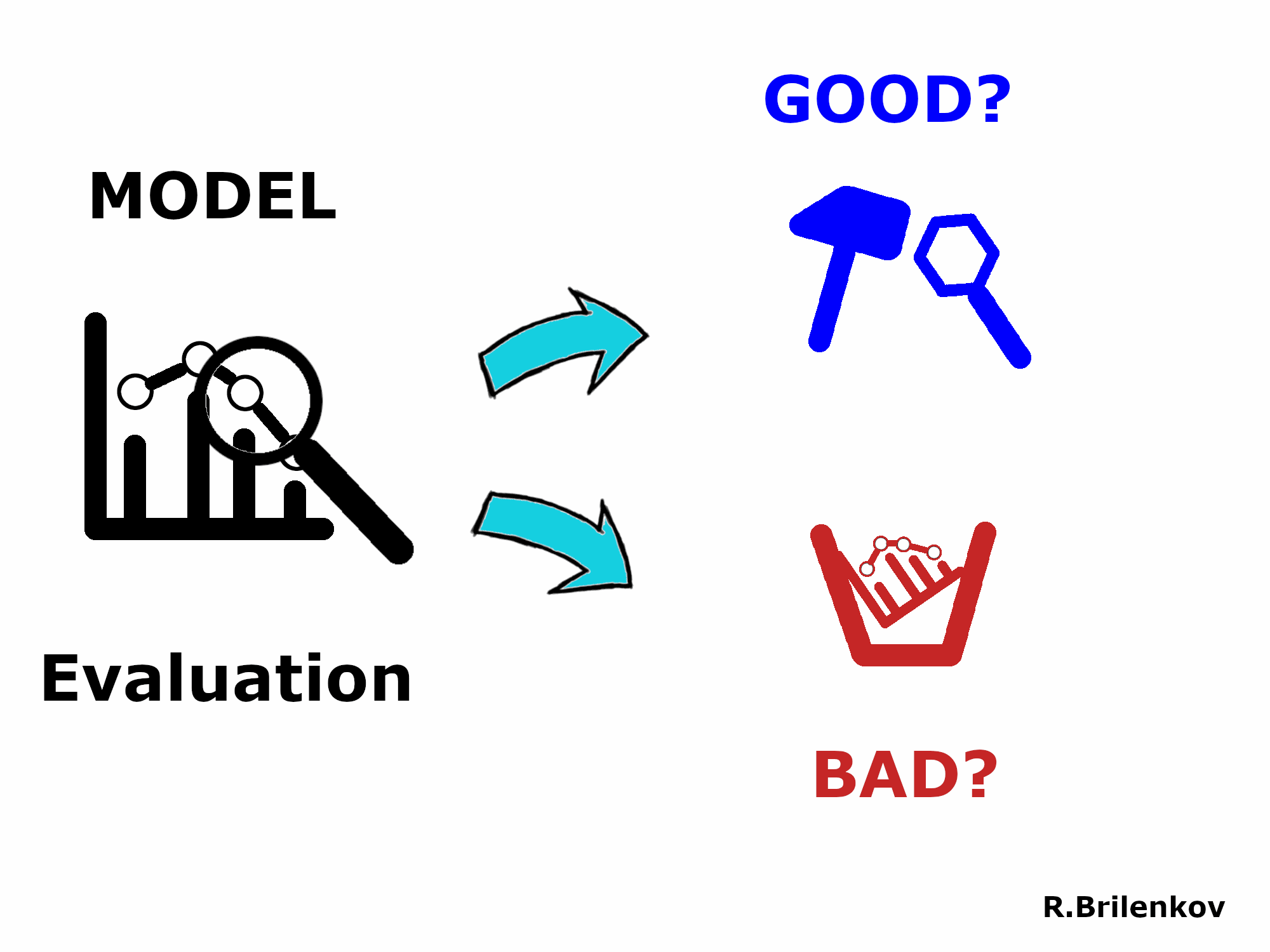## The Most Influential NLP Papers on Google Scholar## Few-shot Learning Explained: Examples, Applications, Research## Zhenghao Xiao

Data science enthusiast

## Choosing the appropriate correlation coefficient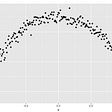## Box-Cox Transformation, Explained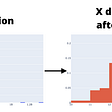## The Roots of Random Forests## Statistical Resampling in Python